Bottle

Bottle with wine costs 58 USD. Wine is 27 USD more expensive than the empty bottle.

How much is the bottle itself?

Result

x =  15.5 USD

Solution:x+y = 58
y = x+ 27

x+y = 58
x-y = -27

x = 312 = 15.5
y = 852 = 42.5

Calculated by our linear equations calculator.

Leave us a comment of this math problem and its solution (i.e. if it is still somewhat unclear...):Be the first to comment!To solve this verbal math problem are needed these knowledge from mathematics:

Do you have a system of equations and looking for calculator system of linear equations?

Next similar math problems:

1. Sweets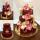3 chocolate and 7 cakes cost 85, - CZK. 2 chocolates and 6 cakes cost 86, - CZK. How much is 5 chocolates and 9 cakes? I wonder how to get the result, but only by logic without the use of a system of equations
2. Money duoJulius and Mark have together 45 euros. Mark has 50% more money than Julius. Determine the amount of money that have Mark and Julius.
3. TheatroTheatrical performance was attended by 480 spectators. Women were in the audience 40 more than men and children 60 less than half of adult spectators. How many men, women and children attended a theater performance?
4. Three daysDuring the three days sold in stationery 1490 workbooks. The first day sold about workbooks more than third day. The second day 190 workbooks sold less than third day. How many workbooks sold during each day?
5. Three friendsThe three friends spent 600 KC in a teahouse. Thomas paid twice as much as Paul. Paul a half less than Zdeněk. How many each paid?
6. Pages of book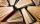Lenka calculated that if every day to read 16 pages of the book, read a book a day earlier than had read only 14 pages a day. How many pages has this book?
7. Magic numberThe number 135 split to two addends so that one addend was 30 greater than 2/5 the addend.
8. Sisters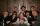Sisters Janka and Danka are saved together 220 CZK. On a trip to Janka wants to take a fifth of her savings and Danka quarter. Then they will have 50 CZK on the trip. How much money saved Janka and Danka?
9. TreesAlong the road were planted 250 trees of two types. Cherry for 60 CZK apiece and apple 50 CZK apiece. The entire plantation cost 12,800 CZK. How many was cherries and apples?
10. Waiting roomIn the waiting room are people and flies. Together they have 15 heads and 50 legs (fly has 6 legs). How many people and flies are in the waiting room?
11. Equations - simpleSolve system of linear equations: x-2y=6 3x+2y=4
12. The dormitoryThe dormitory accommodates 150 pupils in 42 rooms, some of which are triple and some are quadruple. Determine how many rooms are triple and how many quadruples.
13. Tour35 people went on a tour and paid 8530, -. Employees pay 165, and family members 310. How many employees and how many family members went to the tour?
14. Tram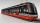In the three-part tram went 206 passengers in front of others, 226 after others and in the middle half of all. How many passengers went in tram total?
15. Two numbersWe have two numbers. Their sum is 140. One-fifth of the first number is equal to half the second number. Determine those unknown numbers.
16. Hotel roomsIn the 45 rooms, there were 169 guests, some rooms were three-bedrooms and some five-bedrooms. How many rooms were?
17. Buttons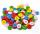Shirt has 6 buttons, blouse has 4 buttons. All buttons are 176. Shirts and blouses are together 36. How many are the shirts and blouses?